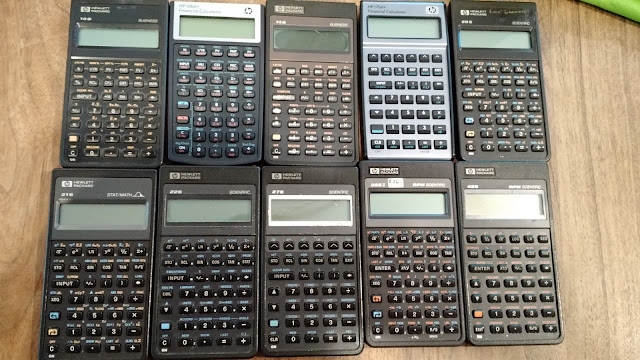## Sunday, July 16, 2017

### Retro Review: HP 22S

Retro Review:  HP 22SHP 22SPioneers:  10B, 10bII+ (updated), 14B, 17bII+ (updated), 20S, 21S, 22S, 27S, 32SII, 42S

Essentials

Company:  Hewlett Packard
Years:  1988 - 1991
Type:  Scientific, Formula Programming
Memory:  371
Operating System: Algebraic
Memory Registers: 26 (A-Z)
Number of Built In Equations: 16

Batteries:  3 LR44, 3 A76, 3 357

Features

The HP 22S is an algebraic calculator that shares the similar style with the HP 20S, 21S, 32S, and 42S.  The top row of keys ([ √x ], [ e^x ], [ LN ], [ y^x ], [ 1/x ], [ Σ+ ]) act not only as function keys but soft menu keys.

The standard variety of functions are present:

* Trigonometric, hyperbolic, exponential, and logarithmic functions
* Conversions:  kg/lb, °C/°F, cm/in, ltr/gal, H/HMS, DEG/RAD
* Probability:  nCr, nPr, n!  (the factorial function only accepts integer)
* Integer part, fractional part, absolute value, round to fix setting
* Statistics:  1 Variable and Linear Regression (y = mx + b)
* Storage arithmetic
* Base conversions: Binary, Decimal, Octal, Hexadecimal.  Decimal mode is floating decimal mode.
* This is really cool:  the rectangular (x-COORD, y-COORD) and polar (RADIUS, ANGLE) conversions get their own keys (well, shifted functions of [ 1 ], [ 2 ], [ 3 ], and [ - ], respectively)
* Percent Change:  old [INPUT] new [(shift)] [1/x] (%CHG)  (nPr and nCr work the same way)

Overall the keys are really easy to work with and the calculator is a pleasure to use.  I like the crisp display.  Plus, if blue is your favorite color like me, the light blue font against the dark background is a plus.

There are two manuals with the HP 22S:  the User Manual, and the excellent Science Student Applications for the HP 22S.

Equations

You can either use one of the 14 built-in equations (listed below) or create your own.  While you are creating your own, you can use either the [STO] or [RCL] keys to call up a letter.  All variables are one letter and global. Equations that are longer than the 12 character display can be scrolled by the [ √x ] and [ Σ+ ] keys.

Equations can be evaluated (evaluate the right side of the equation) or solved (a menu will show the variables to be solved for).  Equations are solved in a reasonably amount of time.

The 16 built in equations, which are displayed by the equations themselves (no titles):
(Source: HP 22S manual)

 Title Equation Length of a Line/Vector R=SQRT(X^2+Y^2+Z^2) Roots of a Quadratic Equation X=(-B+J*SQRT(B^2-4*A*C))÷2÷A Real roots only, J = -1 or 1 Equation of Motion (Position) X=S+V*T+.5*A*T^2 X = position, V = velocity, A = acceleration Equation of Motion (Final Velocity) F=V+A*T Kinetic Energy E=.5*M*V^2 Force Between Two Objects (Physics or Electric Force) F=K*A*B÷R^2 K = 6.67408E-11 m^3 kg^-1 s^-2 (gravitational force) K = 1.602176621E-19 J (electron charge) Joule’s Law P=I^2*R Ideal Gas Law P*V=N*R*T Gibb’s Free Energy G=H-T*S Pressure of a Fluid P=I+D*G*H G = 9.80665 m/s^2 Radioactive Decay -K*T=LN(N÷I) Thin Lens Equation O*F+I*F=O*I Diffraction A=ASIN(M*L÷D) Exponential Growth and Decay Y=F+(I-F)*EXP(K*T) Root Mean Square R=SQRT(Σx^2÷n) Σx^2 and n come from statistical data Time Value of Money (End of Period payments/periods assumed) (P*100÷I-F)*(1+I÷100)^-N-P*100÷I=B N = number of payments/periods B = present value I = periodic interest rate P = payment F = future value

Final Verdict

The HP 22S is a great calculator to own.  I love the rectangular/polar conversion set up.  The display is great.

My only criticism is that I wish the 22S had more memory.  371 bytes can store a handful equations, but data points that are used in the statistical calculations and storing values in the variables A-Z eat up the memory as well.

4 out of 5 stars.

Eddie

This blog is property of Edward Shore, 2017

Coming soon (tentative):  a review of a favorite Texas Instruments calculator, TI-68.

### HP 15C: Error Function and Lower Tail Normal Cumulative Function

HP 15C:   Error Function and Lower Tail Normal Cumulative Function Formulas Used  Error Function  erf(x) = 2 ÷ √π * ∫( e^(-t^2) dt, t = 0 t...